﻿ 大盘牛熊的判别研究及检验 The Research and Examination of Bull Market and Bear Market

Statistics and Application
Vol. 08  No. 04 ( 2019 ), Article ID: 31701 , 15 pages
10.12677/SA.2019.84072

The Research and Examination of Bull Market and Bear Market

Yu Liu, Chunlai Xiao

School of Science, Northern University of Technology, Beijing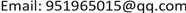Received: Jul. 25th, 2019; accepted: Aug. 7th, 2019; published: Aug. 14th, 2019ABSTRACT

In order to balance the systematic risk in the spot market, investors often choose to hedge the risk through hedging. So accurately judging the bull bear market conversion mechanism in the market has become the key for scholars to explore. According to relevant theories, MACD, TRIX, DMI and MTM indexes are selected in this paper. By adjusting the appropriate parameters, a model is established to judge the conversion between bull and bear market, and a comprehensive index TREND is generated to determine the hedging time in the bear market and the closing time when the bear market ends in the bull and bear transition period, so as to provide a powerful basis for investors to make accurate decisions.

Keywords:Conversion of Bull and Bear Market, Hedging, Closing Time1. 引言

1.1. 研究背景及目的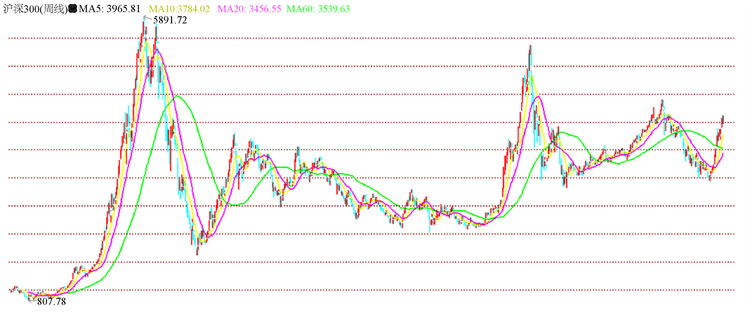Figure 1. 2014~2019.4 Shanghai and Shenzhen 300 stock index price

1.2. 文献综述

2. 原先指标的实际研究

2.1. 技术指标确定

2.1.1. TRIX指标(三重指数平滑移动平均指标)

TRIX指标又叫三重指数平滑移动平均指标，是根据移动平均线理论对一条平均线进行三次平滑处理，再根据这条移动平均线的变动情况来预测股价的长期走势，如图2所示。

TRIX和TRMA两条曲线的相交情况(“金叉”“死叉”)以及这两条曲线的运行方向是该指标的主要研判集中点。TRIX由下往上交叉其平均线时，为长期买进信号；相反，TRIX由上往下交叉其平均线时，为长期卖出信号。

TRIX(27,7)指标是原模型中选取的指标，通过图2来看，TRIX(27,7)指标中“金叉”“死叉”交汇点不明显，存在周期较短，受市场假信号影响较大的问题，据此确定套保及平仓时机存在较大风险。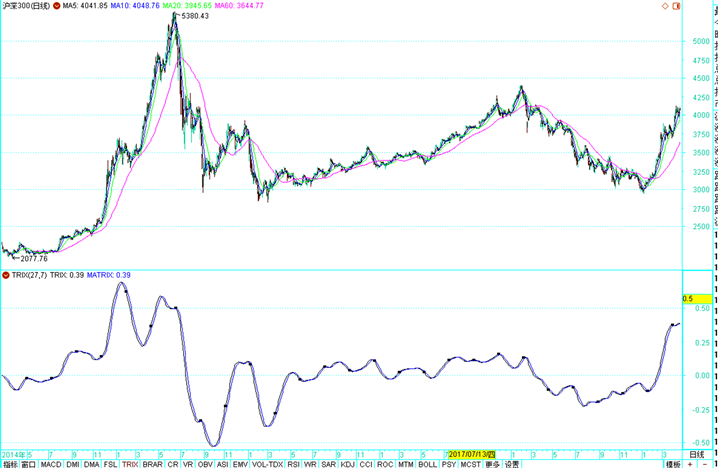Figure 2. TRIX(27,7)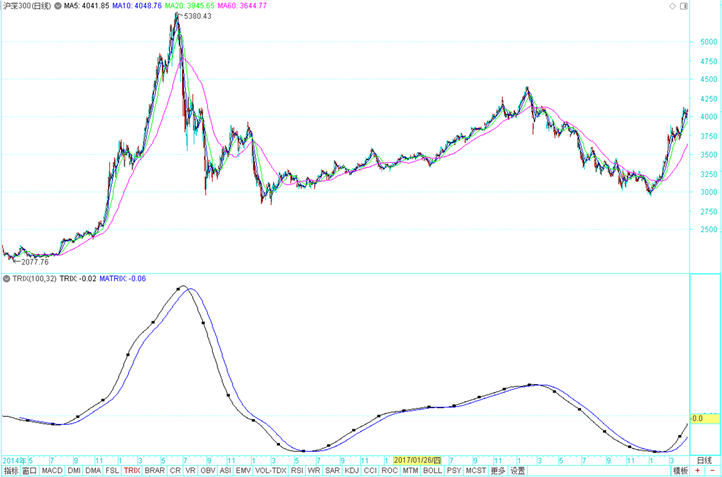Figure 3. TRIX(100,32)

2.1.2. VPT指标(量价曲线)

VPT量价曲线是将量能的增减和股价的涨跌结合起来分析，从而量能的主要运动方向，掌握股价的运行趋势。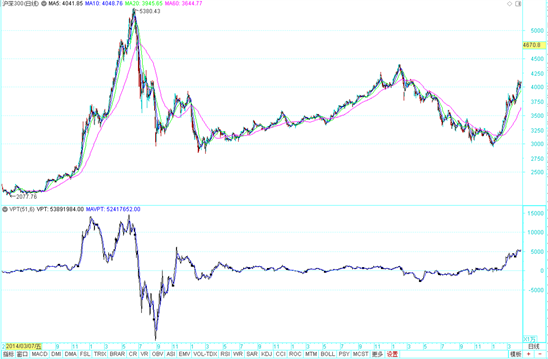Figure 4. VPT(51,6)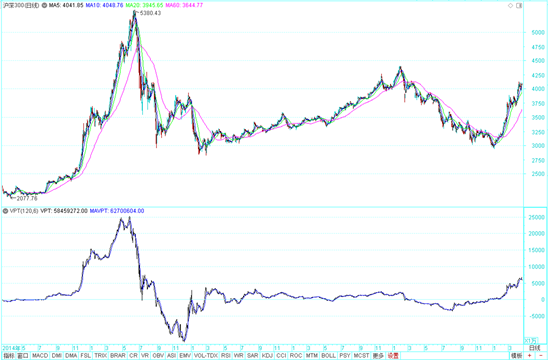Figure 5. VPT(120,6)

2.1.3. DMI指标(动向指标或趋向指标)

DMI指标又叫动向指标或趋向指标，其基本原理是在于寻求股票价格涨跌过程中，股价藉以创新高价或新低价的功能，研判多空力量，进而寻求买卖双方的均衡点及股价在双方互动下波动的循环过程2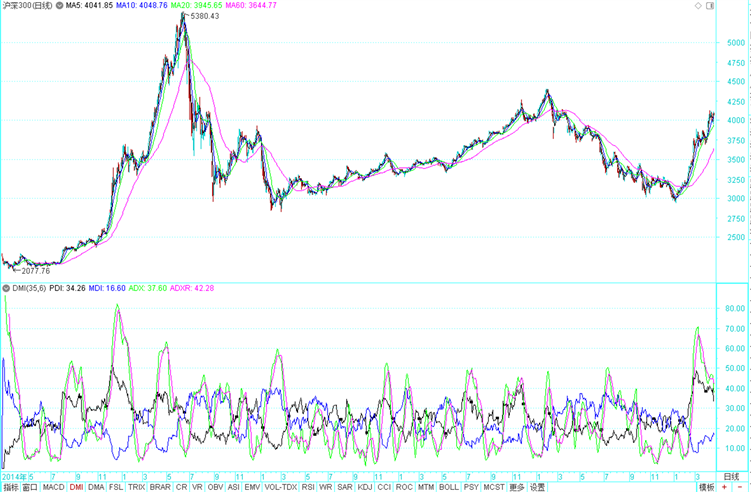Figure 6. DMI(35,6)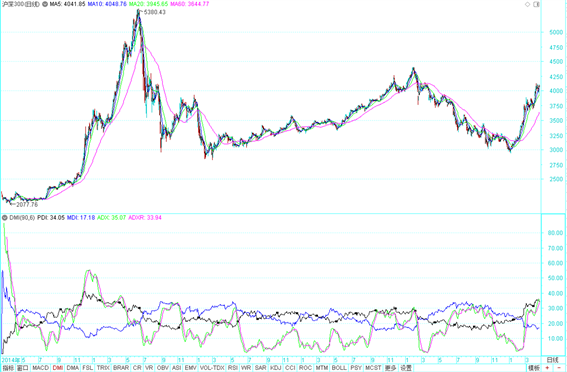Figure 7. DMI(90,6)

2.1.4. MACD指标(指数平滑异同移动平均线)

MACD指标又叫指数平滑异同移动平均线，是运用快速和慢速移动平均线及其聚合与分离的征兆加以双重平滑运算，预测股价中短期趋势3

DIF和MACD的突破与被突破所形成的交叉点被称为“黄金交叉”或“死亡交叉”；当DIF线和MACD线都在0值线以上运行，说明股市处于多头行情，股价将继续上涨，反之为空头行情；柱状线的持续收缩表明趋势运行的强度正在逐渐减弱，当柱状线颜色发生改变时，趋势确定转折。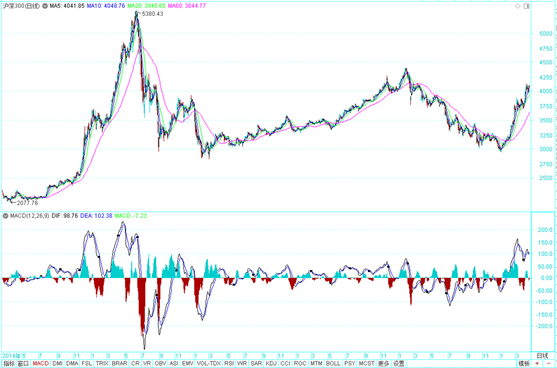Figure 8. MACD(12,26,9)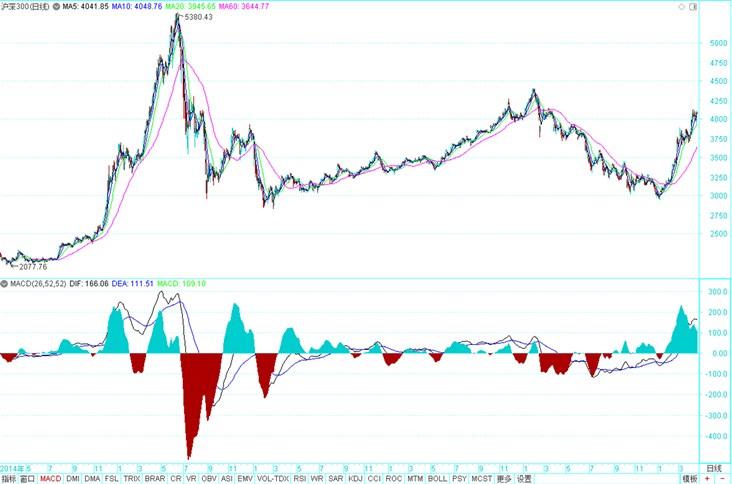Figure 9. MACD(26,52,52)

2.2. 综合指标的构造

$\text{f}\left(\text{TRIX}\left(100,32\right)\right)=\left\{\begin{array}{c}4,\text{TRIX}-\text{TRMA}<0\\ 1,\text{TRIX}-\text{TRMA}>0\end{array}$

$\text{f}\left(\text{VPT}\left(120,6\right)\right)=\left\{\begin{array}{c}4,\text{VPT}<0\\ 1,\text{VPT}>0\end{array}$

$\text{f}\left(\text{DMI}\left(90,6\right)\right)=\left\{\begin{array}{c}4,-\text{DI}>+\text{DI}\\ 1,+\text{DI}>-\text{DI}\end{array}$

$\text{f}\left(\text{MACD}（26,52,52）\right)=\left\{\begin{array}{c}\text{if}\left(\text{and}\left(\text{DIX}<0,\text{DEA}<0,\text{MACD}<0\right),4,3\right)\\ \text{if}\left(\text{and}\left(\text{DIX}>0,\text{DEA}>0,\text{MACD}>0\right),1,2\right)\end{array}$

$\text{MIX}=\text{f}\left(\text{TRIX}\right)+\text{f}\left(\text{VPT}\right)+\text{f}\left(\text{DIM}\right)+\text{f}\left(MACD\right)$

$TREND=\left\{\begin{array}{l}进入熊市，当\text{\hspace{0.17em}}MIX\ge 15\\ 进入牛市，当\text{\hspace{0.17em}}MIX\le 5\end{array}$ (1)

Table 1. TREND indicator calculation results

3. 综合指标改进的实际研究

3.1. MTM指标(动量指标)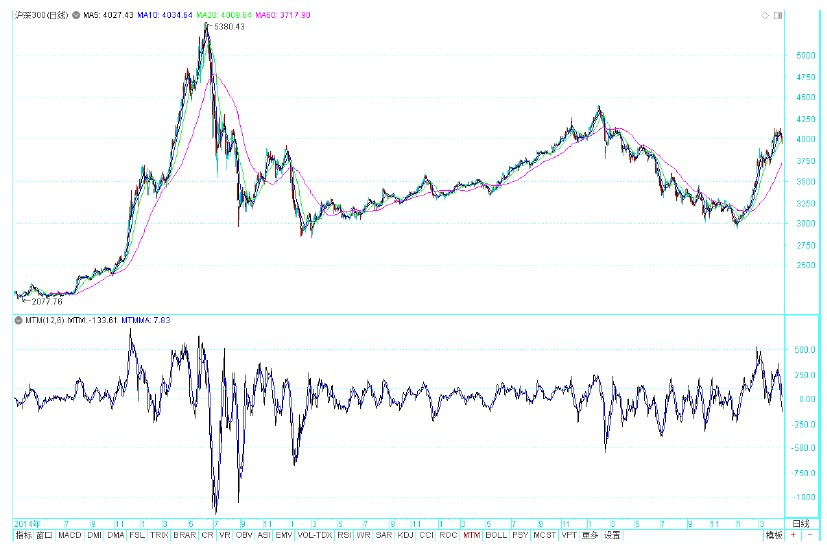Figure 10. MTM(12,6)

3.2. 新综合指标的建立

$\text{f}\left(\text{VPT}\left(120,6\right)\right)=\left\{\begin{array}{c}4,\text{VPT}<0\\ 1,\text{VPT}>0\end{array}$

$\text{f}\left(\text{MTM}\left(94,6\right)\right)=\left\{\begin{array}{c}4,\text{VPT}<0\\ 1,\text{VPT}>0\end{array}$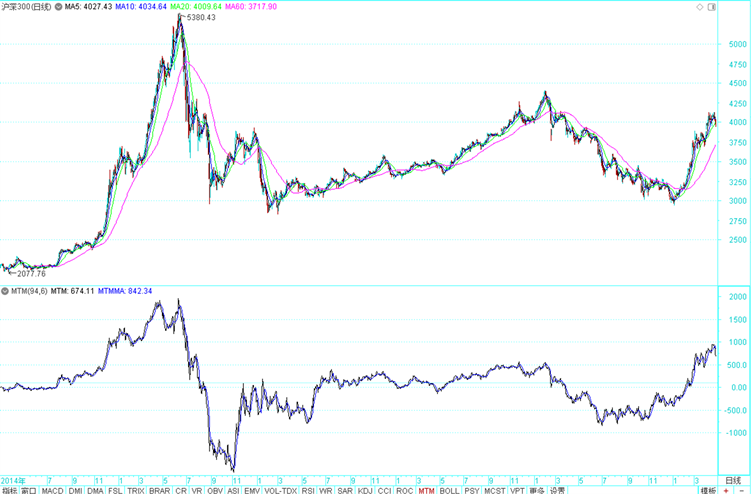Figure 11. MTM(94,6)

$\text{MIX}=\text{f}\left(\text{TRIX}\right)+\text{f}\left(\text{MTM}\right)+\text{f}\left(\text{DIM}\right)+\text{f}\left(MACD\right)$

$\text{TREND}=\left\{\begin{array}{c}进入熊市，当\text{MIX}\ge 15\\ 进入牛市，当\text{MIX}\le 5\end{array}$

Table 2. The coming and ending of the bear market based on comprehensive technical indicators

4. 新综合指标的检验

Table 3. Test results of comparison of new and old indicators

5. 小结

The Research and Examination of Bull Market and Bear Market[J]. 统计学与应用, 2019, 08(04): 631-645. https://doi.org/10.12677/SA.2019.84072

1. 1. 姚芳. 收益率条件分布下的沪深300投资组合研究[D]: [硕士学位论文]. 北京: 北方工业大学, 2012.

2. 2. 刚梦醒. 中国股票市场牛熊转换机制研究[J]. 洛阳理工学院学报(社会科学版), 2012, 27(2): 32-36.

3. 3. 刘建华. 中国股市熊市、牛市的量价关系分析[J]. 漳州师范学院学报(哲学社会科学版), 2008, 22(2): 26-30.

4. 4. 李泽圣. 基于牛市与熊市下的上证指数与深证成指波动溢出效应分析[D]: [硕士学位论文]. 安庆: 安庆师范大学, 2018.

5. NOTES

2DMI指标定义和公式来自姜金胜著的《股票操作指标精萃》P126。

3MACD指标的定义和公式来自姜金胜著的《股票操作指标精萃》P91。